Magic Hexagon
 To the Main Page   "Mathematische Basteleien"

What is a Magic Hexagon?
The magic square first
 ...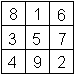... This is certainly well known:  You can distribute the numbers 1 to 9 in a 3x3 square in such way that the sums vertically, horizontally and diagonally have the same value. It is 15.You find more on my page  Magic Square.

 ...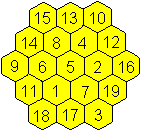... Correspondingly a magic hexagon is a figure, which contains the numbers 1 to 19 and where the sums horizontally (-), sloping up to the right (/) and up to the left  (\) are equal. It is 38.  There is only one hexagon, too.Searching for Magic Hexagons top
Hexagons of  the degree n
The magic hexagon is in a sequence of  increasing hexagons.
 ...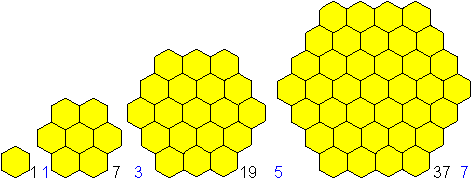... The hexagons have 1,7,19, 37, ... small hexagons.  Generally the hexagon of the order n has 3n²-3n+1 hexagons. The hexagons have 1,3,5,7,..., generally 2n-1, rows.

Derivation of the term 3n²-3n+1
If you calculate the differences of the numbers 1,7,19,37,..., you get 6,12,18, ... and then as the difference of the differences 6, 6, 6, ... .
Thus you have a sequence of the form f(n)=an²+bn+c.
 n=1 makes1=a+b+c  n=2 makes 7=4a+2b+c  n=3 makes 19=9a+3b+c The system of equations has the solution a=3, b= - 3 and c=1. Thus the n-th term of the sequence is 3n²-3n+1.

There is 3n²-3n+1=3n(n-1)+1. This is the idea for the following geometric view.
 ...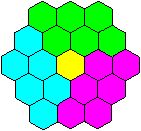... You can divide every hexagon in three parallelograms with the measurements n and (n-1).  A small hexagon is left.  In the drawing is n=3.

Searching for the magic number
There are equal sums in a magic hexagon. This sum H is called the magic number of the hexagon. If you add all numbers in a hexagon, you have the sum S=H+H+...+H (z times) or S=zH. The variable z is the number of parallel rows.

The number of rows is 1, 3, 5, 7, generally z = 2n-1.

According to the sum formula  1+2+3+...+m = m(m+1)/2 you get
S = (3n²-3n+1)[3(n+1)²-3(n+1)+1]/2 = (9n4-18n³+18n²-9n+2)/2.

Then you have H = S/z = S/(2n-1) = (9n4-18n³+18n²-9n+2)/[2(2n-1)] = (9n4-18n³+18n²-9n+2)/(4n-2).
The magic number, given as a quotient, must be an integer.

You can show by a clever calculation that there is the only solution n=3.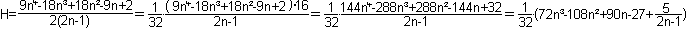You recognize that H or 32H is only a whole number, if 5/(2n-1) is, too. n=3 is a solution that fits. The numbers n= -2, 1,0 don't fit.

You can also find this solution by a computer. You replace n by "all" numbers in the term H=(9n4-18n³+18n²-9n+2)/(4n-2) and find the integers  -2, 1,0, and 3.

Searching for the places of the 19 numbers
 ...... You see that, if there is a magic hexagon, it must have the order 3.  It exists! It is amazing that there is only one magic hexagon. It isn't easy to find the distributions of the numbers 1 to 19. The search is a combination of logic and trial.  You find descriptions at (3), (6), (Torsten Sillke, URL below).To the History of the Magic Hexagon  top
Martin Gardner  made this topic popular like he did with many problems lately.
He tells a nice story in book (4).
He heard of the magic hexagon by a letter sent by Clifford W.Addams, a retired employee at a railway company from Philadelphia. This man started in working on the hexagon in 1917 and found a solution in 1957 by trial and error. He lost it and found it again in 1962.
Gardner highly regarded the problem, when he put the mathematician Charles W. Trigg of the university of Los Angeles on it. He found out that the magic hexagon is unique and obviously unknown in the mathematical literature.
Trigg published his proof in "Recreational Mathematics" (3) in 1964. You can read his article at Torsten Sillke (URL below).

The problem was already known in the end of the 19th century.
You can read at Harvey Heinz (URL below): "Jerry Slocum mailed me a copy of an advertisement (?) dated 1896, crediting W. Radcliffe, Isle of Man, U.K. with this discovery in 1895".

Heinrich Hemme published an article in the magazine Bild der Wissenschaft (1988) and Hans F. Bauch in Wissenschaft und Fortschritt  (1990) that the royal architect (königlicher Baumeister) Ernst von Haselberg from Stralsund knew, solved and proved the definiteness of this problem in 1887 (1), (5), (6).
 ...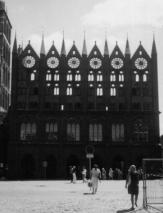... This is a decoration wall beside the town hall of Stralsund, which was renovated during "von Haselberg"'s period of office.  When I visited Stralsund in 1995 I thought it was worth taking a photo.

Magic T-Hexagon top
There is another magic hexagon however formed by triangles. It is called the magic T-hexagon (triangle hexagon). The hexagon above is called H-hexagon (hexagon hexagon) in difference.
 ...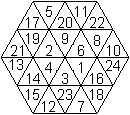... The hexagon is an arrangement of  24  equilateral triangle. You can distribute the numbers 1 to 24, so that it becomes magic.  12 sums horizontally (-), sloping up to the right (/) and up to the left  (\) are equal. It is 38.Number of the triangles
The magic hexagon is in a sequence of increasing hexagons.
 ...... The hexagon of the order n has 6n² triangles.Magic numbers
It is S=zH again.
S = 1+2+3+ ... +6n² = 6n²(6n²+1)/2 = 18n4+3n² and z = 2n makes
H = S/z = (18n4+3n²)/2n =[3n(6n²+1)]/2
The right hand term H is only then an integer, if the numerator is even. The consequence is, n must be an even number.
Thus only the middle one of the three hexagons shown above can be magic.
There are many magic hexagons of this kind.

To the History of Magic T-Hexagons  top
 ...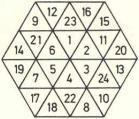... As far as known today the magic T-hexagon was investigated by Hans F. Bauch for the first time. He published his results in the Mathematischen Semesterberichten in 1991 (7). The drawing is an example from the original version, a T-hexagon with a simple inner hexagon.The T-hexagon is called "magic hexagon D(4)". D is for Dreieck (triangle), 4 for the number of rows.

You find the name  T-Hexagon on John Baker's web site (URL below). Obviously John Baker and David King found the T-Hexagons once more,  independent from Hans F. Bauch. You can read on John Baker's Site: "This arrangement was discovered on 13th September, 2003 and as far as we can ascertain is the first example of a magic T-hexagon."

Variants of the Magic Hexagon  top
1) Magic hexagon with integers
 ...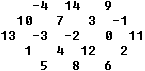... You can find an arrangement of the successive numbers -4, -3, -2, ..., 13, 14  in a hexagon of the order 3, so that it is magic. The magic number is 19.

 ...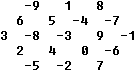... This hexagon contains the numbers  -9  to +9 with the magic number 0.You can probably generalize it: You can find an arrangement of the numbers   -3n(n-1)/2  to  +3n(n-1)/2 in a hexagon of the order 3, so that a magic hexagon with the magic number 0 develops. (Information by Torsten Sillke, URL below).
There is no proof yet.

You find magic hexagons of the orders 4, 5 and 7 at en.wikipedia. They have start numbers >1. Zahray Arsen is the author.

2) Magic hexagon and a mean
 ...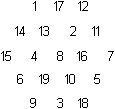... The hexagon of the order 3 contains the numbers 1 to 19 like the magic hexagon above.  All the 15 rows don't have the same sum, but the same mean 10.Origin: Fred W. Helenius ( Torsten Sillke)

3) Magic star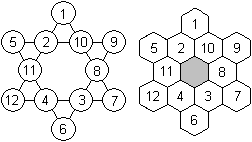It has the numbers 1 to 12. There are 6 equal sums.  The magic number is 26.The magic star was known in the 19th century. There are 96 arrangements in book (2).

You find more on the internet at "Suzanne Alejandre and Mutsumi Suzuki's Magic Stars" (URL below)

4) Hexagram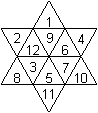It has the numbers 1 to 12. There are 6 sums with 4 summands.  The magic number is 33.Found at MathWorld  (Bolt, B.; Eggleton, R.; and Gilks, J. "The Magic Hexagram." Math. Gaz. 75, 141-142, 1991)

5) First figure of 9 hexagons
 ...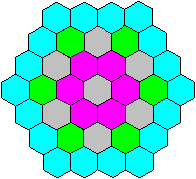...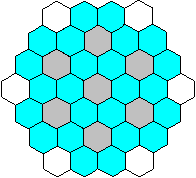... If you take away 7 small hexagons at a hexagon of the order 4, you get a figure with 3 concentric rings and a chain of 6 hexagons.

 ...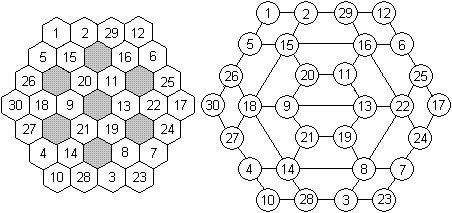... You can distribute the numbers 1 to 30 in this hexagon (2 views) in such a way that it becomes magic.  There are 9 equal sums with 6 summands produced by the numbers in the corners of the small hexagons. The magic number is 93.  There are many solutions.
Origin: Harvey Heinz (URL below)

6) Second figure of 9 hexagons
 ...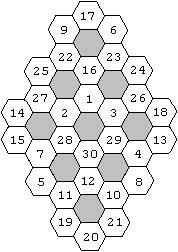... There is another arrangement of 9 hexagons, which you can fill with the numbers 1 to 30, so that it becomes magic.  The figure is formed by 9 rings with common parts.  The magic number is 93. This is the sum of the numbers of one hexagon.  There are many solutions.  I found an information on Jaewook Shin's site with a picture as a reference: "The original source of the problem: Gu-Su-Ryak by Choi, Seok Jung (1646~1715). This book is displayed in the museum of Daejeon history in Daejeon, Korea."
Origin: http://www.mcs.anl.gov/~jaewook/papers/ms_thesis.html  (2006 online)

The Magic Hexagon on the Internet     top

German

Wikipedia
Magisches SechseckErnst von Haselberg

English

David King
Hall of Hexagons

Eric W. Weisstein  (World of Mathematics)
Hexagon, Talisman Hexagon, Magic Hexagon, Magic Hexagram

Frank R. Kschischang;
The Magic Hexagon

Hans F Bauch
The magic hexagon of Ernst v. Haselberg   (.pdf-file)

Mutsumi Suzuki (bei  mathforum)
Magic Stars   (recommended)

Torsten Sillke
Magic Hexagon, Magic-Hexagon-Trigg

Wikipedia
Magic hexagon

References   top
(1) Ernst von Haselberg:
Section 795: Zeitschrift für mathematischen und naturwissenschaftlichen Unterricht 19 (1888) 429     Aufgabe
Section 801: Zeitschrift für mathematischen und naturwissenschaftlichen Unterricht 20 (1889) 263-264 Auflösung
(2) Hermann Schubert: Mathematische Mußestunden, Neubearbeitung von F.Fittig, Walter de Gruyter und Co, 1935
(3) Charles W. Trigg: A Unique Magic Hexagon, Recreational Mathematics Magazine (January-February 1964)
(4) Martin Gardner: Mathematisches Labyrinth, Braunschweig/Wiesbaden 1979 [ISBN 3-528-08402-2]
(5) Heinrich Hemme: Das magische Sechseck, Bild der Wissenschaft (Oktober 1988) 164-166
(6) Hans F. Bauch: Zum magischen Sechseck von Ernst v. Haselberg, Wissenschaft und Fortschritt 40:9 (1990)
(7) Hans F. Bauch: Magische Figuren in Parketten, Mathematische Semesterberichte 38:1 (1991)
(8) Klaus-Peter Rudolph, Hans Friedrich Bauch: Gurami - das neue Zahlenrätsel, Berlin 2011 [ISBN 978-3-9811892-3-0]

Feedback: Email address on my main page